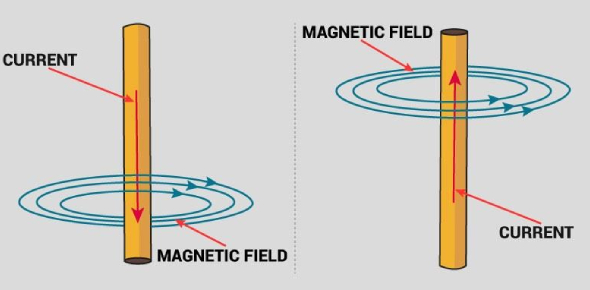# Magnetic Effects Of Electric Current

15 Questions | Total Attempts: 5736SettingsTime: 30 Minute

• 1.
The magnetic field lines produced by a bar magnet:
• A.

Originate from the South pole and end at its North Pole

• B.

Originate from the North pole and end at its East Pole

• C.

Originate from the North Pole and end at its South Pole

• D.

Originate from the South pole and end at its West Pole

• 2.
A soft iron bar is inserted inside a current-carrying solenoid. The magnetic field inside the solenoid:
• A.

Will decrease

• B.

Will increase

• C.

Will become zero

• D.

Will remain the same

• 3.
The north pole of Earth’s magnet is in the:
• A.

Geographical South

• B.

Geographical East

• C.

Geographical West

• D.

Geographical North

• 4.
A current carrying conductor is held in exactly vertical direction. Inorder to produce a clockwise magnetic field around the conductor, the current should be passed in the conductor:
• A.

From top towards bottom

• B.

From left towards right

• C.

From bottom towards top

• D.

From right towards left

• 5.
The force exerted on a current carrying wire placed in a magnetic field is zero when the angle between wire and the direction of magnetic field is:
• A.
• B.
• C.
• D.
• 6.
A car headlamp of 48 W works on the car battery of 12 V. The correct fuse for the circuit of this car headlamp will be:
• A.

3 A

• B.

5 A

• C.

10 A

• D.

13 A

• 7.
An induced current is produced when a magnet is moved into a coil. The magnitude of induced current does not depend on:
• A.

The speed with which the magnet is moved

• B.

The number of turns of the coil

• C.

The resistivity of the wire of the coil

• D.

The strength of the magnet

• 8.
A constant current flows in a horizontal wire in the plane of the paper from east to west as shown below, the direction of magnetic field at a point will be north to south:
• A.

Directly above the wire

• B.

Directly below the wire

• C.

At a point located in the plane of the paper, on the north side of the wire

• D.

At a point located in the plane of the paper, on the south side of the wire

• 9.
If two circular coils can be arrange in any of the tree situations shown in the diagram below, then their mutual induction will be:
• A.

Maximum in situation (a)

• B.

Maximum in situation (b)

• C.

Maximum in situation (c)

• D.

The same in all situations

• 10.
A coil of insulated copper wire is connected to a galvanometer forming a loop and a magnet is: A: Held stationary B: Moved away along its axis C: Moved towards along its axis There will be induced current in:
• A.

A only

• B.

A and B only

• C.

B and C only

• D.

A, B and C

• 11.
An electric current passes through a straight wire in the direction of south to north. Magnetic compasses are placed at points A and B as shown in the figure. What is your observation?
• A.

The needle will not deflect

• B.

Only one of the needles will deflect

• C.

Both the needles will deflect in the same direction

• D.

The needles will deflect in the opposite directions

• 12.
A soft iron bar is introduced inside a current carrying solenoid. The magnetic field inside the solenoid:
• A.

Will become zero

• B.

Will decrease

• C.

Will increase

• D.

Will remain unaffected

• 13.
Which of the following diagrams correctly shows the magnetic field produced by a current-carrying wire?
• A.
• B.
• C.
• D.
• 14.
A 3-pin mains plug is fitted to the cable for a 1kW electric cable to be used on a 250 V ac supply. Which of the following statement is not correct?
• A.

A fuse should be fitted in a live wire

• B.

A 13 A fuse is the most appropriate value to use

• C.

The neutral wire is coloured black

• D.

The green wire should be connected to the earth pin

• 15.
A current flows in a wire running between the S and N poles of a magnet lying horizontally as shown in the figure below: The force on the wire due to the magnet is directed:
• A.

From N to S

• B.

From S to N

• C.

Vertically downwards

• D.

Vertically upwards

Related TopicsBack to top